### Maximum Likelihood Estimation

To estimate the parameters in a linear model with mean functionby maximum likelihood, you need to specify the distribution of the response vector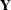. In the linear model with a continuous response variable, it is commonly assumed that the response is normally distributed. In that case, the estimation problem is completely defined by specifying the mean and variance ofin addition to the normality assumption. The model can be written as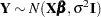, where the notation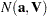indicates a multivariate normal distribution with mean vectorand variance matrix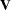. The log likelihood forthen can be written as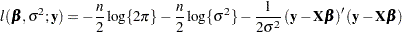This function is maximized inwhen the sum of squares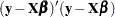is minimized. The maximum likelihood estimator ofis thus identical to the ordinary least squares estimator. To maximize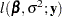with respect to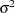, note that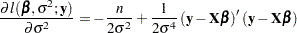Hence the MLE ofis the estimator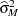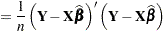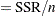This is a biased estimator of, with a bias that decreases with.